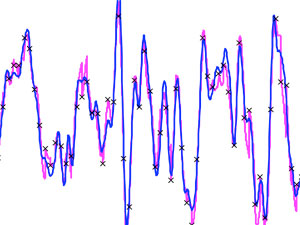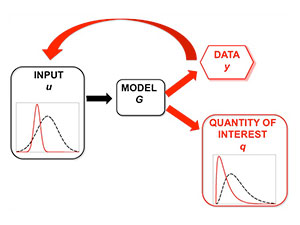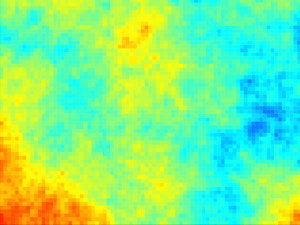# Research## Data Assimilation

A central research challenge for the mathematical sciences in the 21st century is the development of principled methodologies for the seamless integration of (often vast) data sets with sophisticated mathematical models. Such data sets are becoming routinely available in almost all areas of engineering, science and technology as well as in commerce and in the social sciences. More >>## Inverse Problems

A mathematical model for a physical experiment is a set of equations which relate inputs to outputs. Inputs represent physical variables which can be adjusted before the experiment takes place; outputs represent quantities which can be measured as a result of the experiment. The forward problem refers to using the mathematical model to predict the output of an experiment from a given input. The inverse problem refers to using the mathematical model to make inferences about input(s) to the mathematical model which would result in given measured outputs. More >>## Stochastic Modelling

The problems of data assimilation and inverse problems lead naturally to many challenging questions in the area of stochastic modelling. For example random or stochastic differential equations which are invariant with respect to a given probability measure arise in the study of Bayesian inverse problems; the ergodic properties of stochastic dynamical systems, together with properties of the random attractor, characterize data assimilation algorithms. More >>